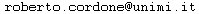# The Quadratic Minimum Spanning Tree Problem (QMSTP)

### The problem

An undirected graph G = (V,E) is given, with a linear cost function c on the edges and a quadratic cost function q on the pairs of edges.

Find a spanning tree of minimum total cost.

### Test instances

Test set employed in R. Cordone and G. Passeri, Solving the Quadratic Minimum Spanning Tree Problem, Applied Mathematics and Computation 218 (23), Pages 11597-11612, August 2012.

#### Description

All instances are in MathProg (i.e. AMPL) format. Their names follow a self-evident convention:

• number of vertices: from 10 to 50 by steps of 5
• density: either 33%, 67% or 100% of the ordered pairs of vertices correspond to edges
• range for the linear costs: either [1;10] or [1;100]
• range for the quadratic costs: either [1;10] or [1;100]
• random seed
• instance number (in each class of size, density and cost structure)

For example: n010d033C010c001Q010q001s-3i1.txt stands for 10 vertices, 33% (10-1)10/2 = 15 edges, linear anche quadratic costs in [1;100]; random seed -3; first instance

### Best known results

The upper bounds have been obtained by Tabu Search QMST-TS on the following benchmarks:

1. Soak et al. (2006)
2. Cordone and Passeri (2008)
3. Oncan and Punnen (2010) (two benchmarks)
4. Sundar and Singh (2010)

The lower bounds have been obtained by branch-and-bound QMST-BB on the following benchmarks:

1. Cordone and Passeri (2008)
2. Oncan and Punnen (2010) (one benchmark)

The computational times refer to a 2.6 GHz Intel Pentium Core 2 Duo E6700 with 2 GB of RAM.Pagina aggiornata il